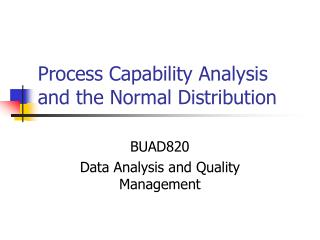DownloadDownload PresentationProcess Capability Analysis and the Normal Distribution

# Process Capability Analysis and the Normal Distribution

Télécharger la présentation## Process Capability Analysis and the Normal Distribution

- - - - - - - - - - - - - - - - - - - - - - - - - - - E N D - - - - - - - - - - - - - - - - - - - - - - - - - - -
##### Presentation Transcript

1. Process Capability Analysis and the Normal Distribution BUAD820 Data Analysis and Quality Management

2. Background • A soda bottle manufacturer wishes to determine process capability of the machine that manufactures soda bottles. To do this, data on an important characteristic of soda bottle, its bursting strength, is collected

3. Raw (sorted) data

4. Normal distribution • Process capability analysis assumes that process data follow the normal distribution • Thus, we should determine whether or not bursting strengths follow the normal distribution • There are several ways to check this assumption

5. Normal distribution check #1: Stem and leaf display Comment: Majority of the data points are in the middle, with few in the tails; distribution appears symmetrical like the normal distribution

6. Normal distribution check #2: Frequency distribution Comment: Data appears to follow the normal distribution

7. Normal distribution check #3: Histogram Comment: Data resemble the normal distribution

8. Normal distribution check #4: Box and whiskers plot Comment: Data resemble the normal distribution

9. Normal distribution check #5: The empirical rule • Bursting strength data have a mean of 264 and standard deviation of 32 • 71 observations are within 1 std. dev. Of the mean (i.e. between 232 and 296) • 94 observations are within 2 std. dev. Of the mean (i.e. between 200 and 328) • All observations are within 3 std. dev. Of the mean (i.e. between 168 and 360)

10. Expected versus observed Comments: The bursting strength data ‘reasonably’ satisfy the empirical rule suggesting that they come from a normal distribution

11. Normal distribution check #6: Normal probability (QQ) plot • This is a more formal test where data are arranged low to high • For each observation, a z-score is calculated • A chart is generated showing data values along Y-axis and Z-scores along X-axis • For data from a normal distribution, the chart should resemble a straight line

12. Normal probability plot (QQ plot) Comments: A reasonably straight line may be drawn through these points, suggesting that the bursting strength data follow normal distribution

13. Normal distribution requirements • In practice, we should be able to make at least a ‘reasonable’ case that bursting strengths follow the normal distribution; a perfect fit is not necessary • Worst case scenario is that the data distribution does not resemble the normal distribution • Process capability analysis should not be used if the data do not reasonably resemble the normal distribution